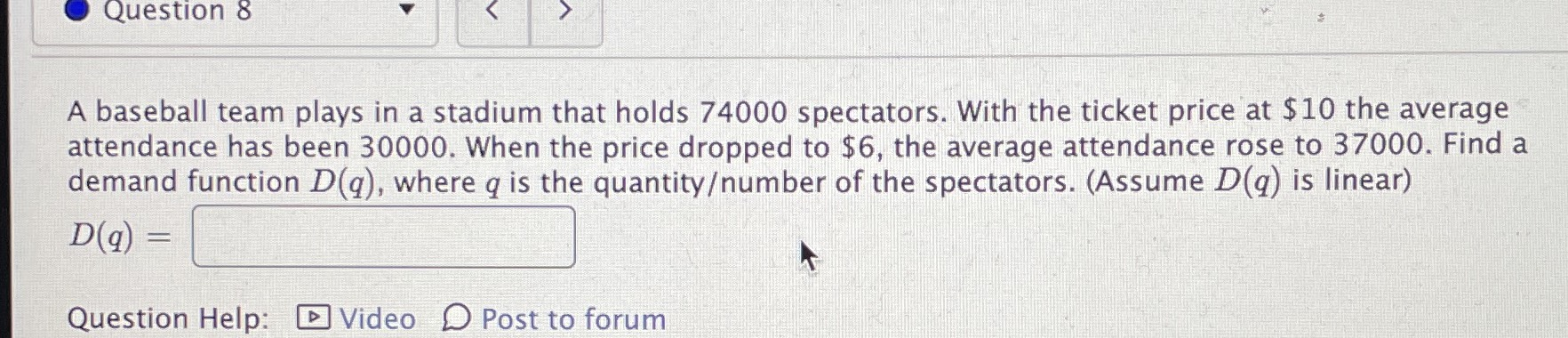### Still have math questions?

Algebra
QuestionA baseball team plays in a stadium that holds $$74000$$ spectators. With the ticket price at $$\ 10$$ the average attendance has been $$30000$$ . When the price dropped to $$\ 6$$ , the average attendance rose to $$37000$$ . Find a demand function $$D ( q ) ,$$ where $$q$$ is the quantity/number of the spectators. (Assume $$D ( q )$$ is linear)

$$D ( q ) = \square$$

$$D(q)= -1750p+ 47500$$